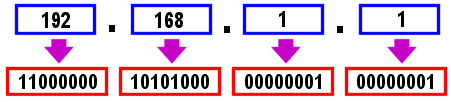# XI. LAN

### How do IP addresses and subnets work?

Products:
All
Keywords:
Fixed IP
Subnet
Subnetting

For a beginner, the concept of subnetting can be bewildering, but don't panic. As a pre-requesiste, you need to be absolultely clear what binary is, and how binary relates to the four octets of an IP address; in this guide, we assume you already are. You also need to understand what 'subnet' means. A subnet, exactly as the name suggests is a subset (a part smaller than the whole) of a larger network which can be specifically identifiable. It is through the use of subnet masks and a network address that we can specifically identify each subnet (a specific range of IP addresses).

The diagram below shows a typical IP address in binary and decimal formats; if you are already confused or do not recognise this type of relationship (base con, we do not recommend that you read on.## Simple Subnets

The Vigor router has a default private address range of 255 possible IP devices running from 192.168.1.1 to 192.168.1.255. Note how the first three octets are always the same and only the fourth octet changes; this is known as a "Class C" subnet. A class C subnet has a subnet mask of 255.255.255.0. If you imagine that in binary, you can see how the mask works :

 Network Address 11000000 10101000 00000001 00000000 =192.168.1.0 Subnet Mask 11111111 11111111 11111111 00000000 =255.255.255.0

Put simply, wherever there is a 1 in the subnet mask, that bit of the network address cannot change and wherebever there is a 0 (zero) in the subnet mask, that bit in the network address can change. On a single user dialup connection to the Internet, with a regular modem, you will receive one public IP address and it will have a subnet mask of 255.255.255.255 - indicating that you have only one address within your subnet.

## More Complicated Subnets

From the above you can see that an octet in the subnet mask of 255 makes things very easy - it means that the corresponding octet in the network address is 'fixed' and having a 0 (zero) means that the corresponding octet in the network address can vary the full range from 1 to 255.

If the subnet mask is 255.255.255.240 (for example), then the subnet will contain 15 addresses because 255 (all bits at 1) minus 240 equals 15. Similarly if the final mask octet is 224, you have a subnet range of 31 addresses (255-224=31), and so on.

If you have an IP address, and its subnet mask, you can calculate which subnet it is within - i.e. the first and last address in that subnet. Here is an example :

 IP Address 213 123 121 119 Subnet Mask 255 255 255 240

The parts of the address masked with 255 are easy to work out, but for the 240 part you need to logically AND (boolean arithmetic) the 240 with the 119. Therefore 11110000 (240) AND 01110111 (119) gives

 Address Octet : 0 1 1 1 0 1 1 1 (119) Mask Octet : 1 1 1 1 0 0 0 0 (240) So Base Address = 0 1 1 1 0 0 0 0 = 112

Another way to calculate the base address is to use a calculator to work out the modulus : e.g. 119 MOD 15 = 112 (Where 15 is already caculated by 255 - mask).

Now we know that the IP address 213.123.121.119 falls within a subnet starting at 213.123.121.112 and includes 15 addresses (up to 213.123.121.126).

## Why is this useful ?

It's useful for lots of reasons, but in the context of your Vigor router, you will want to know the base address in order to set up packet filters. As our example let's say that we wish to block access to a remote site.

## Blocking certain IP addresses within a subnet

As an example, let us assume that you have a Vigor router with a private Class C network behind it; that allows addresses between 192.168.1.1 and 192.168.1.255. You have 50 PCs in total but you want to allow only 20 of them to have Internet access.

Clearly we need to select 20 addresses which we will allow through, and block Internet access to all other addresses within our whole private subnet.

To get 20 addresses, we could use a mask of 235 (255-20) however the Vigor allows you to set masks on 'common' boundaries, so the closes mask to 235 is actually 224. A mask of 224 actually gives us 31 addresses (255-224) (of which we will use 20).

If our PCs are numbered from 192.168.1.10 upwards, let us choose that our

(Note : This document is due to be expanded eventually but there are larger more comprehensive tutorials on IP Addressing elsewhere on the Internet).